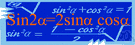# equation

(redirected from equations of the first order)
Also found in: Thesaurus, Medical, Financial, Encyclopedia.

## e·qua·tion

(ĭ-kwā′zhən, -shən)
n.
1. The act or process of equating or of being equated.
2. The state of being equal.
3. Mathematics A statement asserting the equality of two expressions, usually written as a linear array of symbols that are separated into left and right sides and joined by an equal sign.
4. Chemistry A representation of a chemical reaction, usually written as a linear array in which the symbols and quantities of the reactants are separated from those of the products by an arrow or a set of opposing arrows.
5. A complex of variable elements or factors: "The world was full of equations ... there must be an answer for everything, if only you knew how to set forth the questions" (Anne Tyler).

## equation

(ɪˈkweɪʒən; -ʃən)
n
1. (Mathematics) a mathematical statement that two expressions are equal: it is either an identity in which the variables can assume any value, or a conditional equation in which the variables have only certain values (roots)
2. the act of regarding as equal; equating
3. the act of making equal or balanced; equalization
4. a situation, esp one regarded as having a number of conflicting elements: what you want doesn't come into the equation.
5. the state of being equal, equivalent, or equally balanced
6. a situation or problem in which a number of factors need to be considered
7. (Chemistry) See chemical equation
8. astronomy See personal equation

## e•qua•tion

(ɪˈkweɪ ʒən, -ʃən)

n.
1. the act of equating or making equal.
2. the state of being equated or equal.
3. equally balanced state; equilibrium.
4. an expression or a proposition, often algebraic, asserting the equality of two quantities.
5. a symbolic representation in chemistry showing the kind and amount of the starting materials and products of a reaction.
[1350–1400; Middle English < Latin]

## e·qua·tion

(ĭ-kwā′zhən)
1. Mathematics A written statement indicating the equality of two expressions. It consists of a sequence of symbols that is split into left and right sides joined by an equal sign. For example, 2 + 3 + 5 = 10 is an equation.
2. Chemistry A written representation of a chemical reaction, in which the symbols and amounts of the reactants are separated from those of the products by an equal sign, arrow, or a set of opposing arrows. For example, NaOH + HCl = NaCl + H2O is an equation.
ThesaurusAntonymsRelated WordsSynonymsLegend:
 Noun 1equation - a mathematical statement that two expressions are equalmath, mathematics, maths - a science (or group of related sciences) dealing with the logic of quantity and shape and arrangementregression equation, regression of y on x - the equation representing the relation between selected values of one variable (x) and observed values of the other (y); it permits the prediction of the most probable values of ylinear equation - a polynomial equation of the first degreequadratic, quadratic equation - an equation in which the highest power of an unknown quantity is a squarebiquadratic equation, biquadratic - an equation of the fourth degreedifferential equation - an equation containing differentials of a functionsimultaneous equations - a set of equations in two or more variables for which there are values that can satisfy all the equations simultaneouslymathematical statement - a statement of a mathematical relationexponential equation - an equation involving exponential functions of a variable 2 equation - a state of being essentially equal or equivalent; equally balanced; "on a par with the best"status, position - the relative position or standing of things or especially persons in a society; "he had the status of a minor"; "the novel attained the status of a classic"; "atheists do not enjoy a favorable position in American life"egalite, egality - social and political equality; "egality represents an extreme leveling of society"tie - equality of score in a contest 3 equation - the act of regarding as equalequatingequalisation, equalization, leveling - the act of making equal or uniform

## equation

noun the equation between higher spending and higher taxes

## equation

noun
The state of being equivalent:
Translations
مُعادَلةمُعادَلَه
rovnice
ligningregnestykke
yhtälö
egyenletkiegyenlítés
jafnaefnajafna

방정식
rovnica
enačba
ekvation
สมการ
phương trình

## equation

[ɪˈkweɪʒən] N
1. (Math) →
to enter (into) the equation (fig) →
fairness did not seem to enter into the equation
2. (= linking) the equation of sth with sthla identificación de algo con algo

[ɪˈkweɪʒən] n

## equation

n (Math, fig) → Gleichung f; the equation of religion and moralitydie Gleichsetzung von Religion mit Moralität; to balance the equation between work and leisure; that doesn’t even enter the equation

## equation

[ɪˈkweɪʒn] n (Math) →
equations of motion (Phys) →

## equate

(iˈkweit) verb
to regard as the same in some way. He equates money with happiness.
eˈquation (-ʒən) noun
1. a statement that two things are equal or the same. xy+xy=2xy is an equation.
2. a formula expressing the action of certain substances on others. 2H2 + O2 = 2 H2O is an equation.

## equation

rovnice ligning yhtälö jednadžba 等しくすること 방정식 ekvation สมการ phương trình
References in periodicals archive ?
Toutounian, "Convergence analysis of Bernoulli matrix approach for one-dimensional matrix hyperbolic equations of the first order," Computers & Mathematics with Applications, vol.
As a result, for the tetragonal syngony of 4, [bar.4], 4/m classes in case of thermoelastic waves propagation the set of differential equations of the first order takes the following form:
Among the topics are linear partial differential equations of the first order, Laplace and Poisson equations in two dimensions, solving boundary value problems for stationary equations, and diffusion equations in higher dimensions.

Site: Follow: Share:
Open / Close